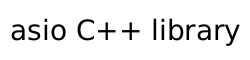#### Resumable C++ 20 Coroutines

NoteThis is an experimental feature.

The `experimental::coro` class provides support for a universal C++20 coroutine. These coroutines can be used as tasks, generators and transfomers, depending on their signature.

```coro<std::string_view> line_reader(tcp::socket stream)
{
while (stream.is_open())
{
std::array<char, 4096> buf;

asio::buffer(buf), deferred);

continue;

co_yield std::string_view { buf.data(), read };
}
}

coro<void, std::size_t> line_logger(tcp::socket stream)
{
while (auto l = co_await reader)
{
std::cout << "Read: '" << *l << "'" << std::endl;
}
}

{
co_spawn(line_logger(std::move(sock),
[](std::exception_ptr, std::size_t lines)
{
std::clog << "Read " << lines << " lines" << std::endl;
}));
}
```

A `coro` is highly configurable, so that it can cover a set of different use cases.

```template<
typename Yield,
typename Return = void,
typename Executor = any_io_executor>
struct coro;
```
###### Yield

The `Yield` parameter designates how a `co_yield` statement behaves. It can either be a type, like `int` or a signature with zero or one types:

```coro<void> // A coroutine with no yield
coro<int> // A coroutine that can yield int

coro<void()> // A coroutine with no yield
coro<int()> // A coroutine that can yield int

coro<int(double)> // A coroutine that can yield int and receive double
```

Receiving a value means that the `co_yield` statement returns a value.

```coro<int(int)> my_sum(any_io_executor)
{
int value = 0;
while (true)
value += co_yield value; //sum up all values
}
```

Putting values into a coroutine can be done it two ways: either by direct resumption (from another coro) or through async_resume. The first value gets ignored because the coroutines are lazy.

```coro<void> c(any_io_executor exec)
{
auto sum = my_sum(exec);
assert(0  == co_await sum(-1));
assert(0  == co_await sum(10));
assert(10 == co_await sum(15));
assert(25 == co_await sum(0));
}

awaitable<void> a()
{
auto sum = my_sum(co_await this_coro::executor);
assert(0  == co_await sum.async_resume(-1, use_awaitable));
assert(0  == co_await sum.async_resume(10, use_awaitable));
assert(10 == co_await sum.async_resume(15, use_awaitable));
assert(25 == co_await sum.async_resume(0, use_awaitable));
}
```
###### `noexcept`

A coro may be noexcept:

```coro<void() noexcept> c;
coro<int() noexcept> c;
coro<int(double) noexcept> c;
```

This will change its @c async_resume signature, from `void(std::exception_ptr)` to `void()` or `void(std::exception_ptr, T)` to `void(T)`. A noexcept coro that ends with an exception will cause `std::terminate` to be called.

Furthermore, calls of `async_resume` and `co_await` of an expired noexcept coro will cause undefined behaviour.

###### Return

A coro can also define a type that can be used with `co_return`:

```coro<void() noexcept, int> c(any_io_executor)
{
co_return 42;
}
```

A coro can have both a `Yield` and `Return` that are non void at the same time.

###### Result

The result type of a coroutine is dermined by both `Yield` and `Return`. Note that in the follwing table only the yield output value is considered, i.e. `T(U)` means `T`.

Table 2. Result type deduction

Yield

Return

`noexcept`

`result_type`

`completion_signature`

`T`

`U`

`false`

```variant<T, U>```

```void(std::exception_ptr, variant<T, U>)```

`T`

`U`

`true`

```variant<T, U>```

```void(variant<T, U>)```

`T`

`void`

`false`

`optional<T>`

```void(std::exception_ptr, optional<T>)```

`T`

`void`

`true`

`optional<T>`

`void(optional<T>)`

`void`

`void`

`false`

`optional<T>`

`void(std::exception_ptr)`

`void`

`void`

`true`

`optional<T>`

`void()`

`void`

`T`

`false`

`optional<T>`

```void(std::exception_ptr, T)```

`void`

`T`

`true`

`optional<T>`

`void(T)`

###### Executor

Every coroutine needs to have its own executor. Since the coroutine gets called multiple times, it cannot take the executor from the caller like an `awaitable`. Therefore a `coro` requires to get an executor or an execution_context passed in as the first parameter.

```coro<int> with_executor(any_io_executor);
coro<int> with_context(io_context &);
```

It is to note, that an execution_context is defined as loosely as possible. An execution context is any object that has a `get_executor()` function, which returns an executor that can be transformed into the executor_type of the coroutine. This allows most io_objects to be used as the source of the executor:

```coro<int> with_socket(tcp::socket);
```

Additionally, a `coro` that is a member function will check the `this` pointer as well, either if it's an executor or an execution context:

```struct my_io_object
{
any_io_executor get_executor();

coro<int> my_coro();
};
```

Finally, a member coro can be given an explicit executor or execution context, to override the one of the object:

```struct my_io_object
{
any_io_executor get_executor();

coro<int> my_coro(any_io_executor exec); // it will use exec
};
```
###### `co_await`

The @c co_await within a `coro` is not the same as `async_resume(deferred)`, unless both coros use different executors. If they use the same, the `coro` will direclty suspend and resume the executor, without any usage of the executor.

`co_await this_coro::` behaves the same as coroutines that use @c asio::awaitable.

###### Integrating with awaitable

As the `coro` member function `async_resume` is an asynchronous operation, it may also be used in conjunction with `awaitable` coroutines in a single control flow. For example:

```#include <asio.hpp>
#include <asio/experimental/coro.hpp>

using asio::ip::tcp;

{
std::string buf;
while (sock.is_open())
{
sock, asio::dynamic_buffer(buf), '\n',
asio::deferred);
co_yield buf.substr(0, n);
buf.erase(0, n);
}
}

asio::awaitable<void> consumer(tcp::socket sock)
{
auto msg1 = co_await r.async_resume(asio::use_awaitable);
std::cout << "Message 1: " << msg1.value_or("\n");
auto msg2 = co_await r.async_resume(asio::use_awaitable);
std::cout << "Message 2: " << msg2.value_or("\n");
}

asio::awaitable<void> listen(tcp::acceptor& acceptor)
{
for (;;)
{
co_spawn(
acceptor.get_executor(),
consumer(co_await acceptor.async_accept(asio::use_awaitable)),
asio::detached);
}
}

int main()
{
asio::io_context ctx;
tcp::acceptor acceptor(ctx, {tcp::v4(), 54321});
co_spawn(ctx, listen(acceptor), asio::detached);
ctx.run();
}
```